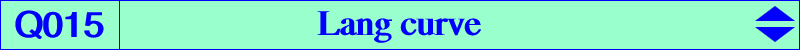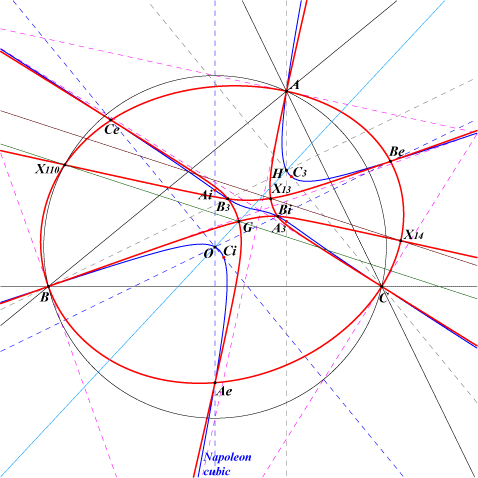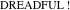X(2), X(11), X(13), X(14), X(110), extraversions of X(11) Ae, Be, Ce, Ai, Bi, Ci : vertices of the Napoleon triangles A3, B3, C3 : intersections of altitudes with the parallel at G to the relative sideline of ABC. (these 9 latter points on the Napoleon cubic)Q015 is the locus of orthopivots of singular orthopivotal cubics. See the FG paper "Orthocorrespondence and orthopivotal cubics" in the Downloads page. It is a 12th degree bicircular curve I call the Lang curve in honour of Fred Lang who kindly gave me the discriminant which allows computation of the equation. For each point P on Q015, the orthopivotal cubic O(P) is either degenerate or has a singularity. See in particular §§6.1, 6.5.1, 6.5.2 in the paper mentioned above. Q015 contains a large number of points on the Napoleon cubic and a total of 18 double points : A, B, C with tangents the bisectors, for which we obtain three strophoids, G with tangents parallel to the asymptotes of the Kiepert hyperbola, the Fermat points X(13), X(14) for which we obtain the strophoids K061a and K061b, X(110), the circular points at infinity, Ae, Be, Ce, Ai, Bi, Ci : vertices of the Napoleon triangles, A3, B3, C3 : intersections of altitudes with the parallels at G. Another remarkable example is K1051 = O(X11).